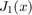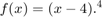# Homework #6

All problems must be in a single *.m file. You may need additional files for functions or class definitions. Make a separate block in the code for each problem. Do not submit individual files for each problem. See previous solutions.

## Problem 1 (1 Point)

Use the symbolic toolkit to calculate the golden ratio used in the golden section search method.

## Problem 2 (1 Points)

Create an animation showing how the golden section search method updates its intervals as it converges on the function minimum.

## Problem 3 (1 Point)

The Rayleigh resolution criterion is defined as the seperation between two objects when the maximum of the Airy pattern from one object is positioned at the first minimum of the Airy pattern in the second object. The Airy pattern has its fisrt mininum whenhas its first zero crossing (other than at x=0). Use the secant method to find this crossing.

## Problem 4 (1 Point)

Create a function that performs the matrix multiplication A*B and returns the result. Your function must use various checks and error handling to anticipate all possible misuse of the function. No credit if we can find a way to break it (get to a MATLAB generated error) or to get the wrong answer.

## Problem 5 (1 Point)

Calculate the first 100 digits of the constant. Save your result in a 1x100 vector.

## Problem 6 (1 Point)

Use Newton's method to find the minimum of the function:## Problem 7 (1 Point)

Use the 'publish' functionality to produce a PDF version of your homework. Submit the PDF to learn as another file. It doesn't have to look pretty. +0.5 extra credit if it looks beautiful and clearly shows the results of your homework solutions.